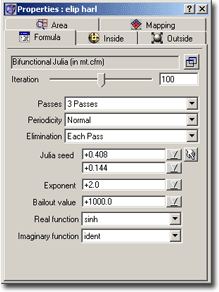### Escapetime Fractals – ParametersFig.: Parameter Window

The Escapetime parameter window has five different tabs.

• The area tab lets you define the base area of the complex number plane. For more information see:Area Window. The “standard” Area window contains more values to adjust. The Escapetime fractal type has transformations, and these formulas provide the additional parameters (and many more…).
• The transformation tab lets you add transformations of the area defined before. For more information about transformation formulas and their meaning see Transformations.You add transformations by double clicking onto a transformation formula in the compiler window or by selecting a transformation formula in the compiler window and pressing the “Add Transformation” image button in the parameter window.
Because a transformation simply “transforms” (or, in other words, takes a complex number and produces a complex number), it is possible to apply several transformations: The successing transformation takes as input the output from the preceding transformation.
And this indeed makes sense: Suppose you have a simple transformation which just “moves” the input area (takes the input value and adds a complex number). Suppose you also have a transformation which does some rotation. If you now want to combine these two transformations you could write a more complicated transformation which “moves” and “rotates”. But wouldn’t it be easier, if you just apply the “movement” transformation, and after that apply the “rotation” transformation? ChaosPro allows you to do that: You can write many simple understandable transformations. And the user who simply wants to create fractals can combine any number of transformations to obtain interesting sets of the complex number plane. For more information about setting parameters of your transformations see Setting Formula Parameters.

One note: Transformations can skrew up the zoom feature: As the zoom feature simply zooms into the area defined in the area tab and transformations “transform” that area ChaosPro is not always able to do a correct zooming. The error occurs if at least one transformation depends on a value which is not fix between zooms.

• The formula tab lets you specify the iteration formula to use for the fractal. Assign such a formula by double clicking onto the formula in the compiler window or by selecting it and pressing the “Set”-button.
You then can change the parameters of this formula.
For more information about setting parameters of your formula see Setting Formula Parameters.

You can set some standard parameters for your formula as the number of iterations, the number of draw passes and the periodicity check logic. If you encounter strange calculation errors I recommend to set “Periodicity Check” to “Off” as it may produce uncorrect results.

• The inside tab lets you choose a coloring method for the inner region of the set (i.e. the area where the maximum number of iterations has been reached). For more information about coloring formulas and their meaning see Coloring Formulas.

In addition to choosing the formula you can set some standard parameters. Although the exact meaning is different, the basic meaning is the same as in the old Colormapping Window. You can specify a solid color for the inside region using the small image button.
For more information about setting parameters of your coloring formula see Setting Formula Parameters.

• The outside tab (which looks like the inside tab) lets you choose a coloring method for the outer region of the set (i.e. the area where the maximum number of iterations has not been reached). For more information about coloring formulas and their meaning see Coloring Formulas.

In addition to choosing the formula you can set some standard parameters. Although the exact meaning is different, the basic meaning is the same as in the old Colormapping Window. You can specify a solid color for the outside region using the small image button. Please note that there are two solid colors, one for the inner region and one for the outer region. If the transformation sets a pixel to “solid” then always the outer solid color is taken.
For more information about setting parameters of your coloring formula see Setting Formula Parameters.